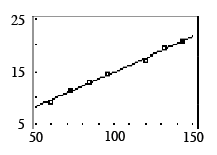### Home > INT1 > Chapter 4 > Lesson 4.2.1 > Problem4-62

4-62.

Robbie’s class collected the following view tube data in problem 4-1.

Distance from wall (in)

Width of field of view (in)

$144$

$20.7$

$132$

$19.6$

$120$

$17.3$

$96$

$14.8$

$84$

$13.1$

$72$

$11.4$

$60$

$9.3$

checksum $\it 708$

checksum $\it 106.2$

1. Use your calculator to make a scatterplot and graph the least squares regression line (LSRL). Sketch the graph and LSRL on your paper. Remember to put a scale on the $x$-axis and $y$-axis of your sketch. Write the equation of the LSRL rounded to four decimal places.

See scatterplot below. $y = 1.6568 + 0.1336x$2. Using your calculator, determine the residuals. Make a table with the distance from wall (inches) as the first column and residual (inches) in the second column. What is the sum of the squares of the residuals?

• See table below.
Use your calculator to find the sum of the squares of the residuals.

 Distance from wall (in) Residual (in) 144 -0.198 132 0.305 120 -0.391 96 0.316 84 0.219 72 0.123 60 -0.374